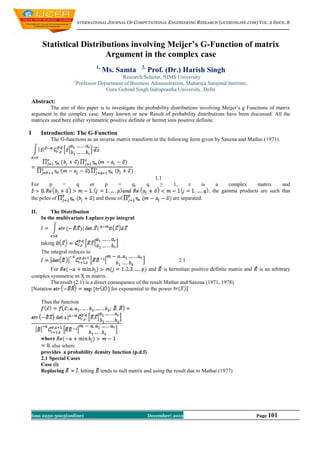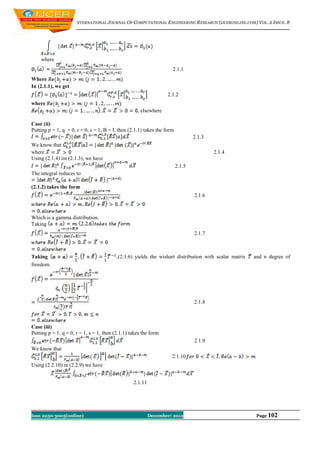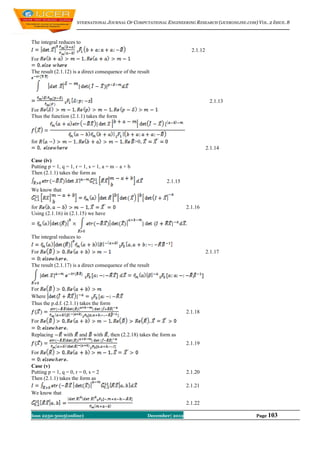Diese Präsentation wurde erfolgreich gemeldet.

# International Journal of Computational Engineering Research(IJCER)

Anzeige
Anzeige
Anzeige
Anzeige
Anzeige
Anzeige
Anzeige
Anzeige
Anzeige
Anzeige
AnzeigeAnzeige
×

1 von 5 Anzeige

# International Journal of Computational Engineering Research(IJCER)

International Journal of Computational Engineering Research (IJCER) is dedicated to protecting personal information and will make every reasonable effort to handle collected information appropriately. All information collected, as well as related requests, will be handled as carefully and efficiently as possible in accordance with IJCER standards for integrity and objectivity.

International Journal of Computational Engineering Research (IJCER) is dedicated to protecting personal information and will make every reasonable effort to handle collected information appropriately. All information collected, as well as related requests, will be handled as carefully and efficiently as possible in accordance with IJCER standards for integrity and objectivity.

Anzeige
Anzeige

### International Journal of Computational Engineering Research(IJCER)

1. 1. INTERNATIONAL JOURNAL OF COMPUTATIONAL ENGINEERING RESEARCH (IJCERONLINE.COM) VOL. 2 ISSUE. 8 Statistical Distributions involving Meijer’s G-Function of matrix Argument in the complex case 1, 2, Ms. Samta Prof. (Dr.) Harish Singh 1 Research Scholar, NIMS University 2 Professor Department of Business Administration, Maharaja Surajmal Institute, Guru Gobind Singh Indraprastha University, Delhi Abstract: The aim of this paper is to investigate the probability distributions involving Meijer’s g Functions of matrix argument in the complex case. Many known or new Result of probability distributions have been discussed. All the matrices used here either symmetric positive definite or hermit ions positive definite. I Introduction: The G-Function The G-functions as an inverse matrix transform in the following form given by Saxena and Mathai (1971). 1.1 For p < q or p = q, q ≥ 1, z is a complex matrix and the gamma products are such that the poles of and those of are separated. II. The Distribution In the multivariate Laplace type integral taking The integral reduces to 2.1 For and is hermitian positive definite matrix and is an arbitrary complex symmetric m X m matrix. The result (2.1) is a direct consequence of the result Mathai and Saxena (1971, 1978). [Notation for exponential to the power Thus the function where else where provides a probability density function (p.d.f) 2.1 Special Cases Case (i) Replacing letting tends to null matrix and using the result due to Mathai (1977) Issn 2250-3005(online) December| 2012 Page 101
2. 2. INTERNATIONAL JOURNAL OF COMPUTATIONAL ENGINEERING RESEARCH (IJCERONLINE.COM) VOL. 2 ISSUE. 8 where 2.1.1 Where In (2.1.1), we get 2.1.2 where elsewhere Case (ii) Putting p = 1, q = 0, r = 0, s = 1, B = I, then (2.1.1) takes the form 2.1.3 We know that where 2.1.4 Using (2.1.4) iin (2.1.3), we have 2.1.5 The integral reduces to (2.1.2) takes the form 2.1.6 Which is a gamma distribution. Taking 2.1.7 Taking (2.1.6) yields the wishart distribution with scalar matrix and n degree of freedom. 2.1.8 Case (iii) Putting p = 1, q = 0, r = 1, s = 1, then (2.1.1) takes the form 2.1.9 We know that 2.1.10 Using (2.2.10) in (2.2.9) we have 2.1.11 Issn 2250-3005(online) December| 2012 Page 102
3. 3. INTERNATIONAL JOURNAL OF COMPUTATIONAL ENGINEERING RESEARCH (IJCERONLINE.COM) VOL. 2 ISSUE. 8 The integral reduces to 2.1.12 For The result (2.1.12) is a direct consequence of the result 2.1.13 For Thus the function (2.1.1) takes the form for >0, 2.1.14 Case (iv) Putting p = 1, q = 1, r = 1, s = 1, a = m – a + b Then (2.1.1) takes the form as 2.1.15 We know that for 2.1.16 Using (2.1.16) in (2.1.15) we have The integral reduces to For 2.1.17 The result (2.1.17) is a direct consequence of the result For Where Thus the p.d.f. (2.1.1) takes the form 2.1.18 For Replacing with and with then (2.2.18) takes the form as 2.1.19 For Case (v) Putting p = 1, q = 0, r = 0, s = 2 2.1.20 Then (2.1.1) takes the form as 2.1.21 We know that 2.1.22 Issn 2250-3005(online) December| 2012 Page 103
4. 4. INTERNATIONAL JOURNAL OF COMPUTATIONAL ENGINEERING RESEARCH (IJCERONLINE.COM) VOL. 2 ISSUE. 8 For Making use of (2.1.22) in (2.1.21) we get 2.1.23 Thus the p.d.f. (2.2.1) takes the form 2.1.24 For Case (vi) Putting p = 1, q = 1, r = 1, s = 2, then (2.1.1) takes the form 2.1.25 We know that 2.1.26 2.1.27 For Using (2.1.26) in (2.1.27) we get 2.1.28 2.1.29 For The result (2.2.29) is a direct consequence of the result 2.1.30 For Then the p.d.f. (2.1.30) takes the form as 2.1.31 Where For Replacing with - , (2.1.31) takes the form 2.1.32 Where For We know that 2.1.33 Making use of (2.2.33) in (2.2.32), we get 2.1.34 Where Issn 2250-3005(online) December| 2012 Page 104
5. 5. INTERNATIONAL JOURNAL OF COMPUTATIONAL ENGINEERING RESEARCH (IJCERONLINE.COM) VOL. 2 ISSUE. 8 We know that the Kummer transformation as 2.1.35 Making use of (2.2.35) in (2.2.34) we get 2.1.36 When Where Case (vii) Putting p = 1, q = 2, r = 2, s = 2, a = -c1, b = -c2, c = a-m, a = -b The (2.1.1) takes the form 2.1.37 Who know that? 2.1.38 For Making use of (2.2.38) in (2.2.37), we get 2.1.39 The result (2.1.39) is a direct consequence of the result 2.1.40 Thus the p.d.f. (2.1.2) takes the following form 2.1.41 Where For Replacing with and with , (2.2.40) reduces to 2.1.42 Where Making use of (2.1.33) in (2.1.42), we get Here, References  Mathai AM.1997. Jacobeans of matrix Transformations and function of Matrix Argument world Scientific Publishing Co. Pvt ltd.  Mathai Am and Saxena R.K. 1971. Meijer’s g Function with matrix argument, Acta, Mexicans ci-tech, 5, 85-92.  R Muirrhead RJ Aspects of multi Variate Statical theory, wicley, New york, 1983. Issn 2250-3005(online) December| 2012 Page 105